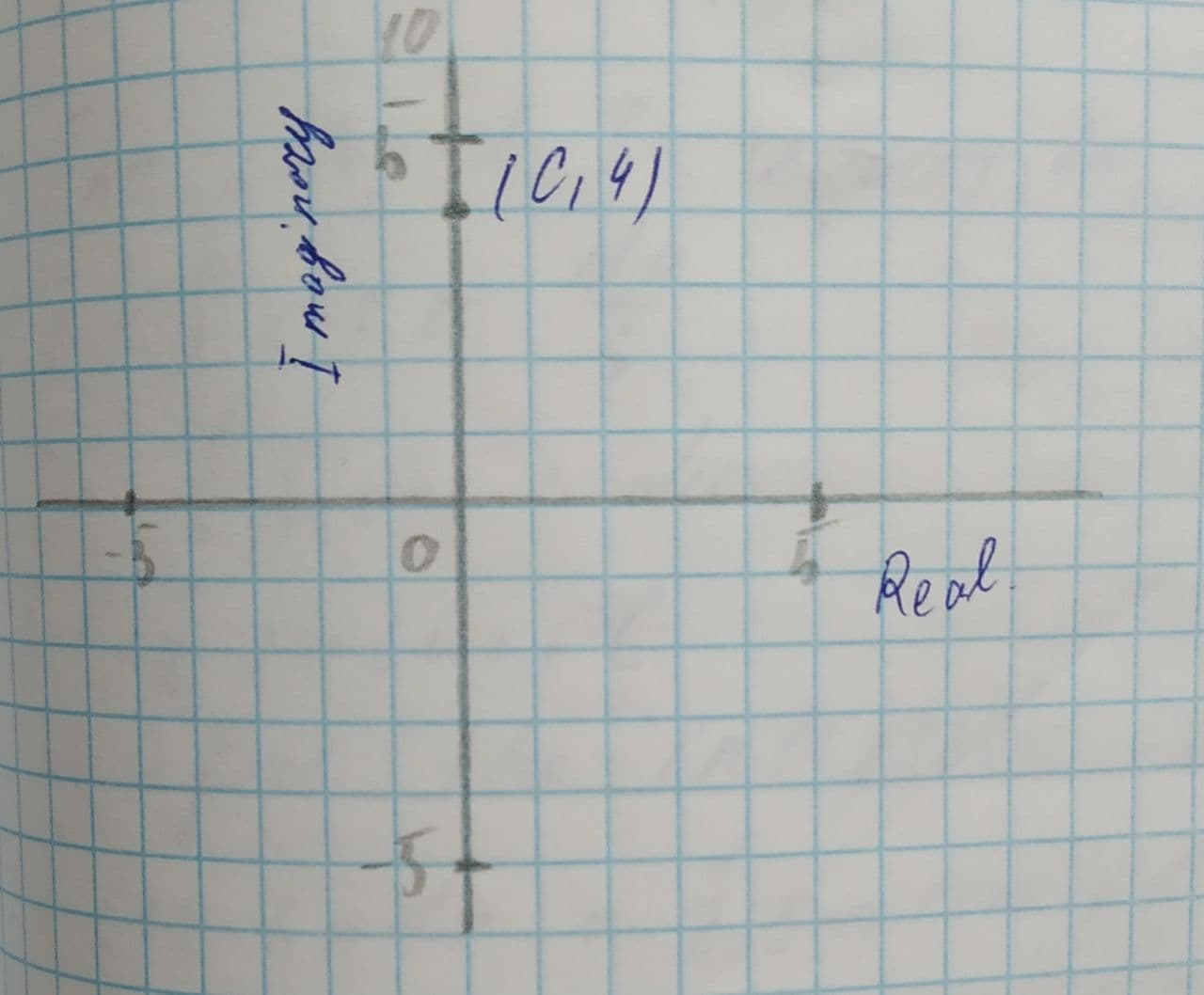Question# Plot a complex number 4i and graph. Specify the absolute value of the number.

Functions
ANSWEREDPlot a complex number graph. Specify the absolute value of the number.
4i2021-08-05

Step 1
The given expression is 4i.
The Cartesian point in complex plane is (0,4).
The graph is shown below:Step 2
The distance formula for points on the Cartesian plane:$$\displaystyle{d}=\sqrt{{{\left({x}_{{{2}}}-{x}_{{{1}}}\right)}^{{{2}}}+{\left({y}_{{{2}}}-{y}_{{{1}}}\right)}^{{{2}}}}}$$.
$$\displaystyle{d}=\sqrt{{{\left({0}-{0}\right)}^{{{2}}}+{\left({0}-{4}\right)}^{{{2}}}}}$$
$$\displaystyle=\sqrt{{{0}+{16}}}$$
=4
The absolute value of the number 4i is 4.